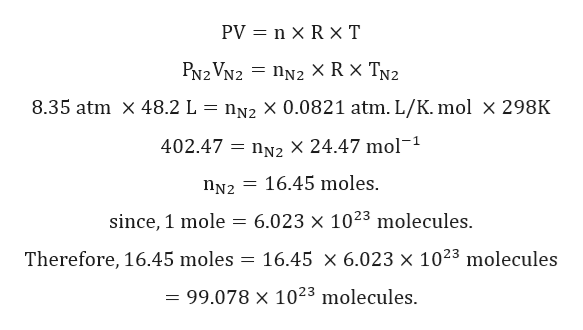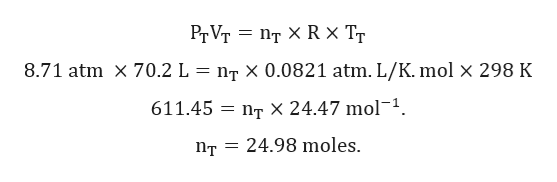138. You have two rigid gas cylinders. Gas cylinder A has a volume of 48.2 L and contains N2(g) at 8.35 atm at 25 °C. Gas cylinder B has a volume of 22.0 L and contains He(g) at 25 °C. When the two cylinders are connected with a valve of negligible volume and the gases are mixed, the pressure in each cylinder becomes 8.71 atm. (Assume no reaction when the gases are mixed.)a. How many nitrogen molecules are present?b. What is the total number of moles of N2(g) and He(g) present after the gases are mixed?c. What was the beginning pressure of cylinder B containing only the He(g) (i.e., before the valve was connected)?d. Think about the He(g) before and after the cylinders were connected. Graph the relationship between pressure and volume (without numbers) for the He(g) showing this change, and explain your answer, making sure to address the variables P, V, n, and T.

Question

138. You have two rigid gas cylinders. Gas cylinder A has a volume of 48.2 L and contains N2(g) at 8.35 atm at 25 °C. Gas cylinder B has a volume of 22.0 L and contains He(g) at 25 °C. When the two cylinders are connected with a valve of negligible volume and the gases are mixed, the pressure in each cylinder becomes 8.71 atm. (Assume no reaction when the gases are mixed.)

a. How many nitrogen molecules are present?

b. What is the total number of moles of N2(g) and He(g) present after the gases are mixed?

c. What was the beginning pressure of cylinder B containing only the He(g) (i.e., before the valve was connected)?

d. Think about the He(g) before and after the cylinders were connected. Graph the relationship between pressure and volume (without numbers) for the He(g) showing this change, and explain your answer, making sure to address the variables P, V, n, and T.

Step 1

Given:

Volume of gas (VN2) = 48.2 L.

Pressure of gas (PN2) = 8.35 atm.

Temperature of gas (TN2) = 25℃ = 298 K.

Volume of gas (VHe) = 22.0 L.

Temperature of gas (THe) = 25℃ = 298 K.

Total pressure when gasses are mixed = 8.71 atm.

Step 2

Calculation for number of molecules of N2 in cylinder A:help_outlineImage TranscriptionclosePV n x R xT PN2 VN2 nN2 X R X TN2 8.35 atm X 48.2 L nN2 X 0.0821 atm. L/K. mol x 298K 402.47 nN2 X 24.47 mol-1 16.45 moles. nN2 6.023 x 1023 molecules since, 1 mole 16.45 x 6.023 x 1023 molecules Therefore, 16.45 moles = 99.078 x 1023 molecules. fullscreen
Step 3

Calculation for total number of moles:

After mixing both the gases:

PT = 8.71 atm.

VT = VN2 + VHe = 48.2 +22.0 = 70.2 L.

TT...help_outlineImage TranscriptionclosePr Vт — Пт XR х T nт х0.0821 atm. L/K. molx 298 K 8.71 atm X 70.2 L 611.45 E Пт X 24.47 mol-1. 24.98 moles Пт fullscreen

Want to see the full answer?

See Solution

Want to see this answer and more?

Our solutions are written by experts, many with advanced degrees, and available 24/7

See Solution
Tagged in

States of matter